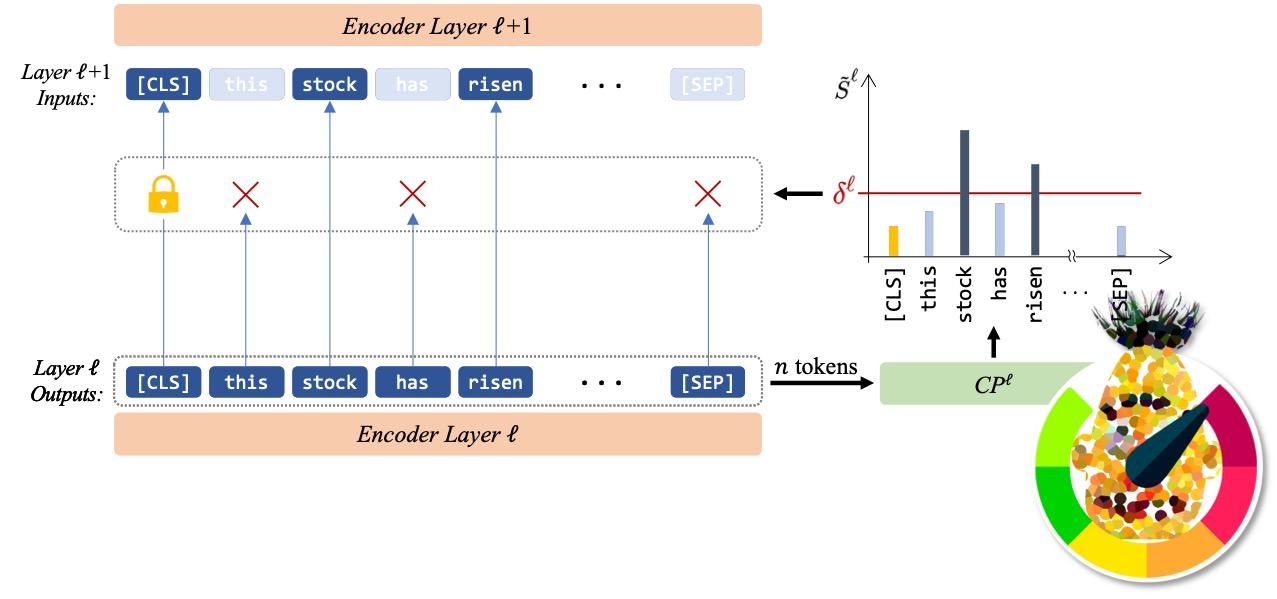# AdapLeR: Speeding up Inference by Adaptive Length Reduction

- 8 mins

This is a post for the ACL 2022 paper AdapLeR: Speeding up Inference by Adaptive Length Reduction.

We propose a novel approach for reducing the computational cost of BERT with minimal loss in downstream performance. AdapLeR dynamically eliminates less contributing tokens through layers, resulting in shorter lengths and consequently lower computational cost. Our contributions are as follows:

• We couple a simple Contribution Predictor (CP) with each layer of the model to estimate tokens’ contribution scores to eliminate redundant representations.
• Instead of an instant token removal, we gradually mask out less contributing token representations by employing a novel soft-removal function.
• We also show the superiority of our token selection strategy over the other widely used strategies by using human rationales.

## Efficient Methods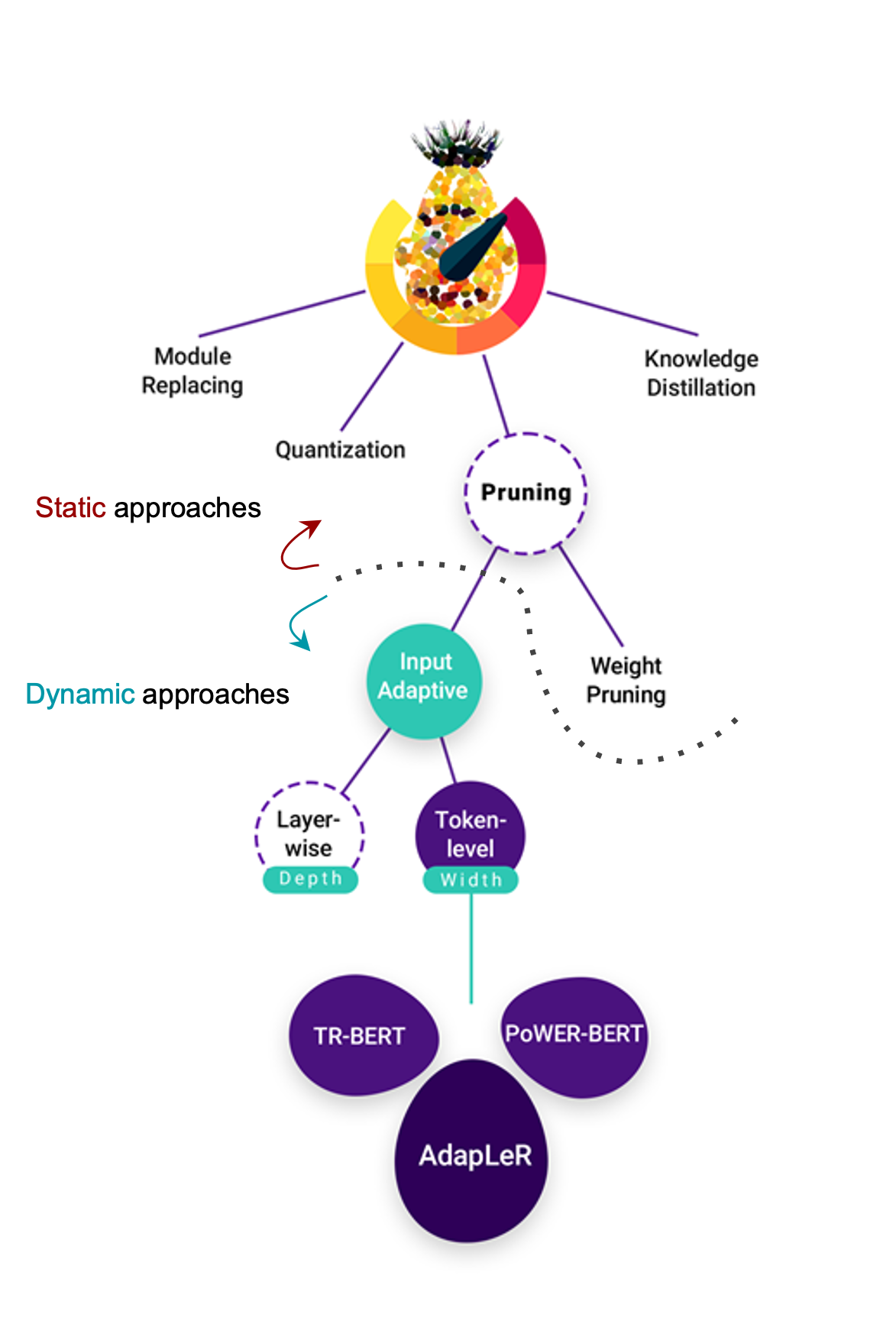There have been various efforts at improving the efficiency of BERT-based models such as Knowledge Distilation, Quantization, Weight Pruning and progressive Module Replacing. Despite providing significant reductions in model size, these techniques are generally static at inference time, i.e., they dedicate the same amount of computation to all input examples, irrespective of their difficulty.

Another branch of pruning methods known as input adaptive pruning aims to address this issue by allowing an input example to exit without passing through all layers or dropping some token representations at inference time. The latter can be viewed as a compression method from a width perspective and it is particularly promising as recent attribution analysis studies have shown that some token representations carry more task-specific information than others, suggesting that only these hidden states could be considered through the model. Moreover, in contrast to layer-wise pruning, token-level pruning does not come at the cost of reducing the model’s capacity in complex reasoning which generally happens at the deeper layers.

In this post, we introduce Adapler, an input-adaptive pruning method and we show the superiority of our method over other state-of-the-art length reduction techniques.

In AdapLeR, each layer dynamically eliminates less contributing tokens. This leads in shorter lengths and as a result, reduced computational cost.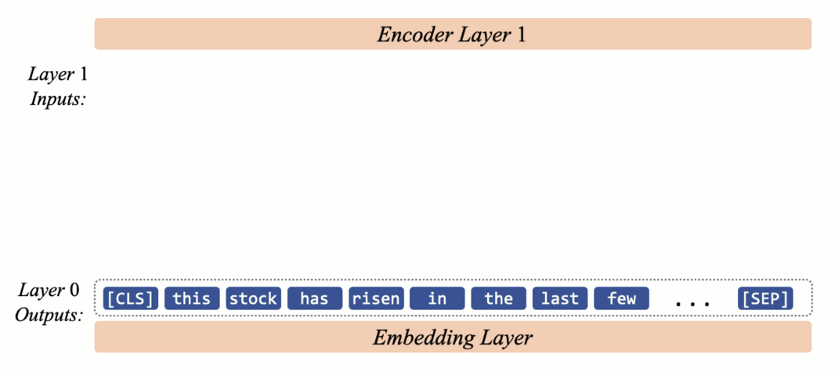The goal is to reduce the number of token representations in a progressive manner

## Inference

In our approach, we eliminate less contributing token representations before delivering them to the next encoder. Therefore in each layer, first a Contribution Predictor (CP) provides an estimation of each tokens’ importance and then a trainable threshold drops the less contributing tokens.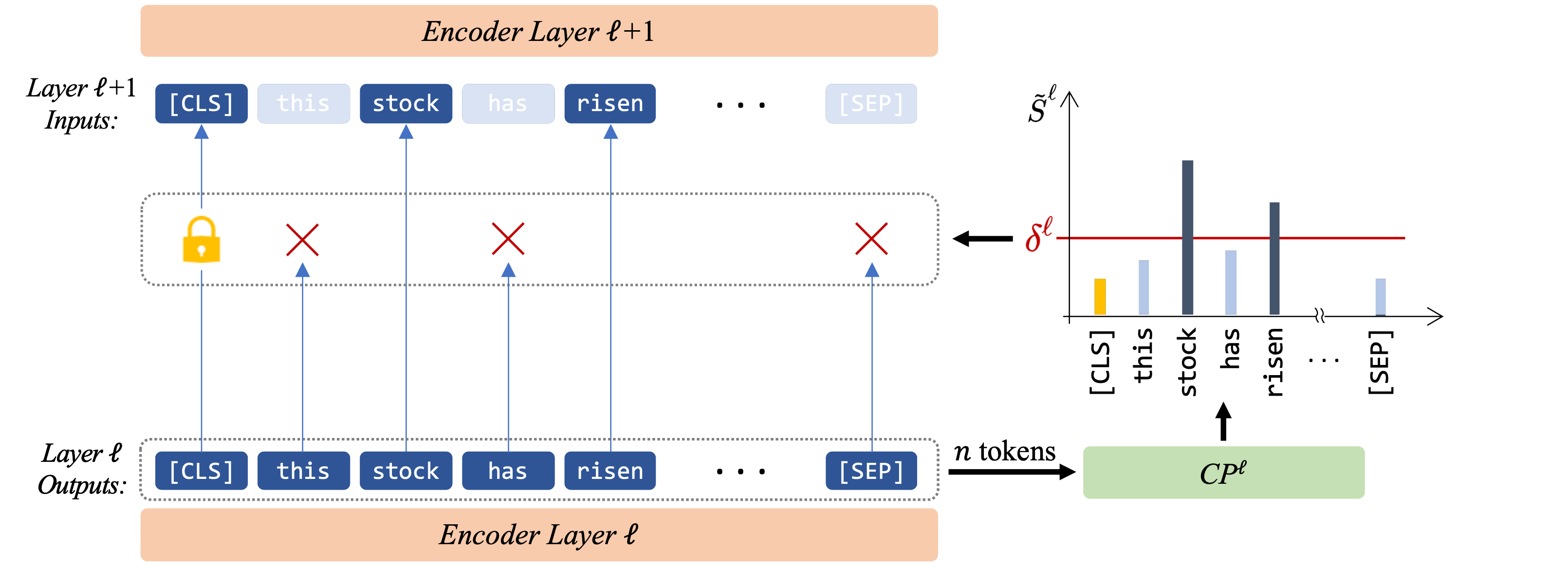### CP

An important step in detecting less contributing tokens is to estimate the importance of each token. we simply add a CP after each layer $$\ell$$ in the model to estimate contribution score for each token representation, i.e., $$\tilde{S}^\ell$$. The model then decides on the tokens that should be passed to the next layer based on the values of $$\tilde{S}^\ell$$. CP computes $$\tilde{S}^\ell$$ for each token using an MLP followed by a softmax activation function.

### Token Removal

After the CP computed the contribution scores, a trainable threshold ($$\delta$$) separates the remaining tokens from the dropping ones. As the sum of the contribution scores is equal to one, a uniform level indicates that all tokens contribute equally to the prediction and should be retained. On the other hand, the lower-scoring tokens could be viewed as unnecessary tokens if the contribution scores are concentrated only on a subset of tokens. Hence, $$\delta$$ has a value equal or smaller than a uniform-level ($$\frac{1}{n}$$) score. Note that the final classification head uses the last hidden state of the $$\text{[CLS]}$$ token. So we preserve this token’s representation in all layers.

## Training

This section breaks down the training procedure into three main challenges: training the CPs alongside the model, removing tokens gradually, and the speedup tuning objective.

### CP Training

For training the CPs, we opted to use saliency scores, which have been recently shown as a reliable criterion in measuring token contributions. The CPs are trained using the KL-divergence of each layer’s CP output with saliency scores extracted from a model finetuned for the given target task.The main training objective is jointly training the CPs with the rest of the model alongside with the target task labels:

$$\mathcal{L}_{\text{CP}}=\displaystyle\sum_{\ell=0}^{L-1}(L-\ell)D_{KL}(\hat{S}^\ell || \tilde{S}^\ell)$$ $$\mathcal{L}=\mathcal{L}_{\text{CE}}+\gamma\mathcal{L}_{\text{CP}}$$

### Soft-Removal

During training, if tokens are immediately dropped similarly to the inference mode, the effect of dropping tokens cannot be captured using a gradient backpropagation procedure. Using batch-wise training in this scenario will also be problematic as the structure will vary with each example.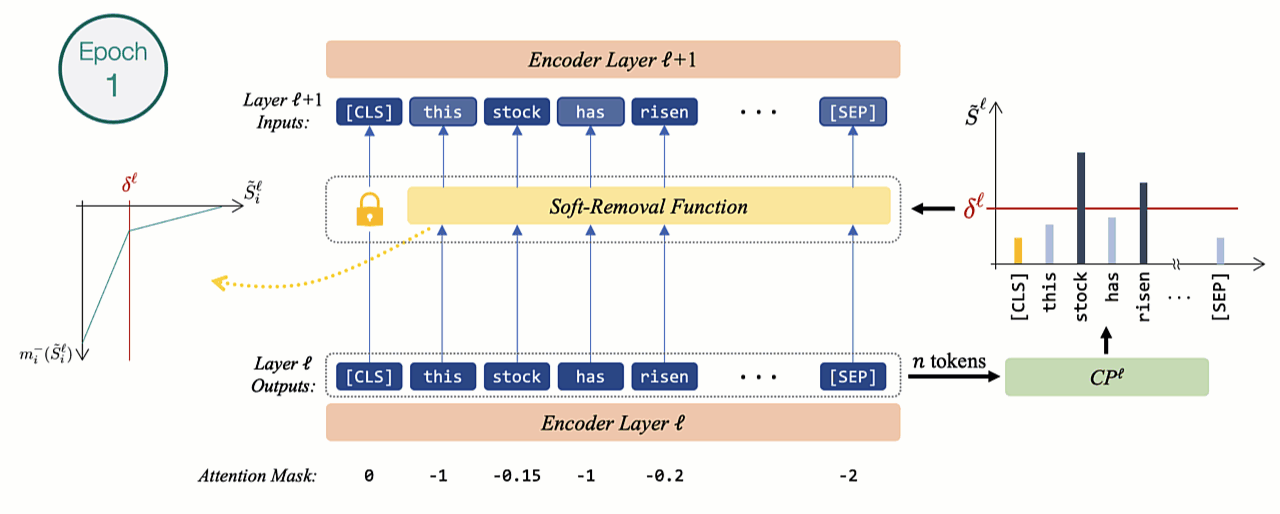Hence, inspired by the padding mechanism of self-attention models we gradually mask out less contributing representations by employing a novel soft-removal function. The less important tokens with scores lower than the threshold ($$\delta$$) are assigned higher negative masking as they get more distant from $$\delta$$. After each epoch, the slope increases exponentially, gradually approaching a hard-masking characteristic similar to inference mode.

### Speedup Tuning

To control the speedup-performance tradeoff we define a separate objective by combining the the cross-entropy loss of the target classification task with a length loss summed over all layers.Note that this objective is responsible for training the thresholds ($$\delta$$) while the previously mentioned loss in “CP Training” section, trains the whole model (except $$\delta$$).

## Results

This Table shows performance and speedup for AdapLeR and other comparison models across eight various text classification datasets: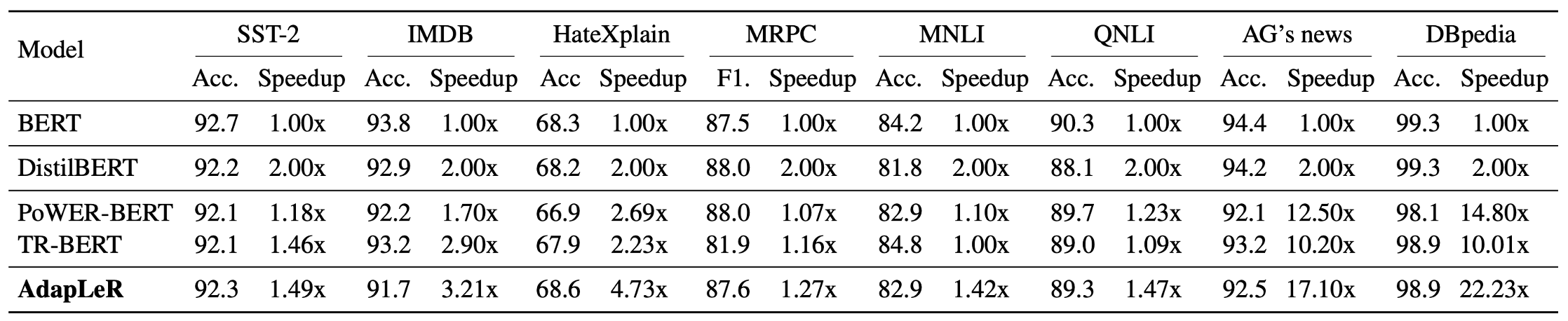As our baseline, we report results for the pre-trained BERT model which is also the backbone of AdapLeR. We also compare against three other approaches: DistilBERT as a static compression method, PoWER-BERT and TR-BERT as two strong length reduction methods. The model’s speedup is defined as the total FLOPs (i.e., the number of floating-point operation) measured on BERT (our baseline) divided by the corresponding model’s total FLOPs. This allows us to assess models’ speedups independently of their operating environment (e.g., CPU/GPU).

Results also reveal some form of dependency on the type of the tasks. Some tasks may need less amount of contextualism during inference and could be classified by using only a fraction of input tokens. For instance, in AG’s News, the topic of a sentence might be identifiable with a single token (e.g., soccer → Topic: Sports).

PoWER-BERT adopts attention weights in its token selection which requires at least one layer of computation to be determined, and TR-BERT applies token elimination only in two layers to reduce the training search space. In contrast, our procedure performs token elimination for all layers of the model, enabling a more effective removal of redundant tokens.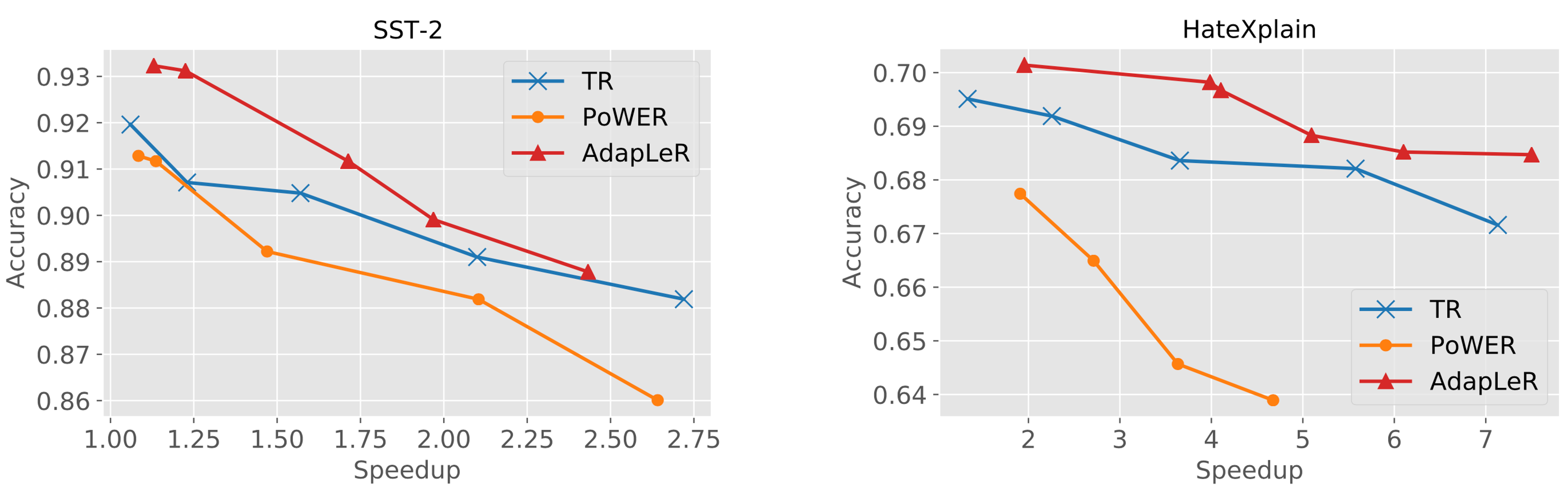Speedup-performance tradeoff curves also show that AdapLeR significantly outperforms the other state-of-the-art length reduction methods.

## Analysis

In this section, we evaluate the behavior of Contribution Predictors (CPs) in identifying the most contributing tokens in the AdapLeR. We resort to human rationale as a reliable upper bound for measuring token importance (see the paper for details).

### Qualitative AnalysisThis Figure illustrates two examples from the SST-2 and QNLI datasets in which CPs identify and gradually drop the irrelevant tokens through layers, finally focusing mostly on the most important token representations; pedestrian (adjective) in SST-2 and tesla coil in the passage part of QNLI (both of which are highly aligned with human rationale).

### Quantitative Analysis

To investigate the effectiveness of trained CPs in predicting human rationales we computed the output scores of CPs in AdapLeR for each token representation in each layer. We also fine-tuned a BERT model on the Movie Review dataset and computed layer-wise raw attention, attention rollout, and saliency scores for each token representation. In addition to these soft scores, we used the uniform-level threshold (i.e., 1/n) to reach a binary score indicating tokens selected in each layer.

As for evaluation, we used the Average Precision (AP) and False Positive Rate (FPR) metrics by comparing the remaining tokens to the human rationale annotations. The first metric measures whether the model assigns higher continuous scores to those tokens that are annotated by humans as rationales. Whereas, the intuition behind the second metric is how many irrelevant tokens are selected by the model to be passed to subsequent layers. We used soft scores for computing AP and binary scores for computing FPR.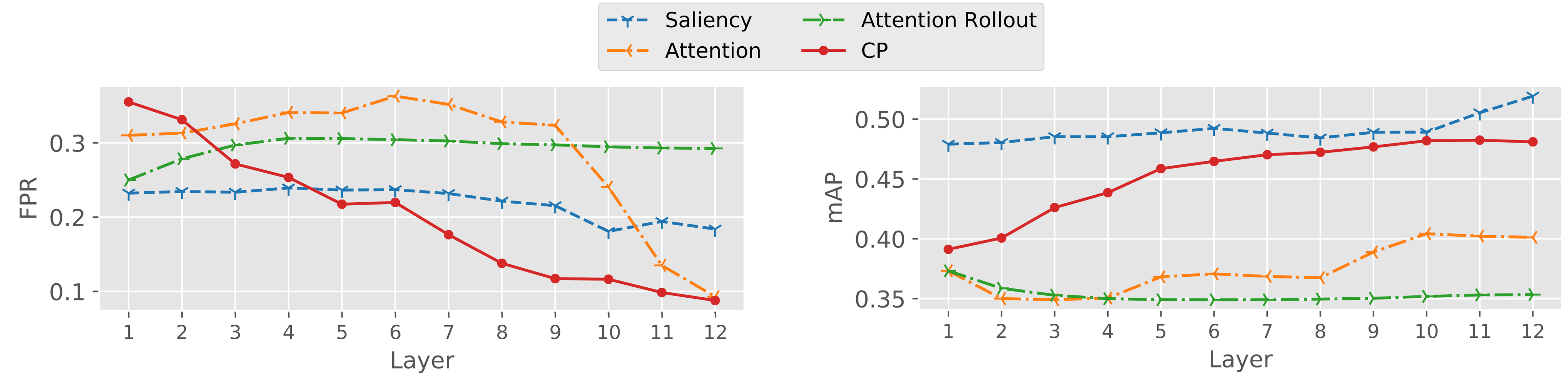This Figure shows the agreement between human rationales and the selected tokens based on the two metrics. In comparison with the other widely used strategies for selecting important tokens, such as salinecy and attention, our CPs have significantly less false positive rate in preserving rationales through layers. Despite having similar FPRs at the final layer, CP is preferable to attention in that it can better identify rationales at the earlier layers, allowing the model to combine the most relevant token representations when building the final one. As a by-product of the contribution estimation process, our trained CPs are able to generate these rationales at inference without the need for human-generated annotations.